×

Search anything:

# Understanding Inception-ResNet V1 architecture

#### Machine Learning (ML)Get this book -> Problems on Array: For Interviews and Competitive Programming

In this article, we have explored the architecture of Inception-ResNet v1 model and understand the need for the model.

1. Introduction to Network Topologies
2. Brief summary of Inception networks
3. Inception-ResNet v1
4. Implementation of Operations in Inception-ResNet v1

Pre-requisites:

# Introduction to Network Topologies

In the early stages of Artificial Intelligence, convolution neural networks (CNN) were just stacked up layers of nodes to create a model. To improve the performance of learning models, data scientists would just stack layers of nodes deeper and deeper. However, this was too computationally expensive, time consuming and prone to errors (which can go undetected). So, scientists and researchers began to develop CNN classifiers and topologies to tackle these precise issues.

One of these classifiers was the Inception network, which was complex and heavily engineered so that its usage would not be nearly as complex. The nature of the network improves performance in terms of speed and accuracy and the chance of errors are greatly reduced. Evolution in the field led to more improved versions of the Inception network which were all iterative improvements on the preceding ones.

Some versions of the Inception networks are as follows:

• Inception v1
• Inception v2
• Inception v3
• Inception v4
• Inception-ResNet

In this article we will discuss the nature of the Inception-RestNet network.

# Brief summary of Inception networks

I have to state that, since the versions are iterative improvements on the previous ones, understanding the newer version will be better when you understand the older one and the upgrades made on it. Links to the research on each network will be listed at the end of this article for reference.

Generally, the introductory version of v1 has filters of multiple convolution sizes (i.e. 1x1, 3x3, 5x5, 7x7 etc.), working together on one layer. The computational cost of this could be reduced if the data from the previous layer passed through a 1x1 filter before going into a 3x3, or 5x5 or 7x7 filter.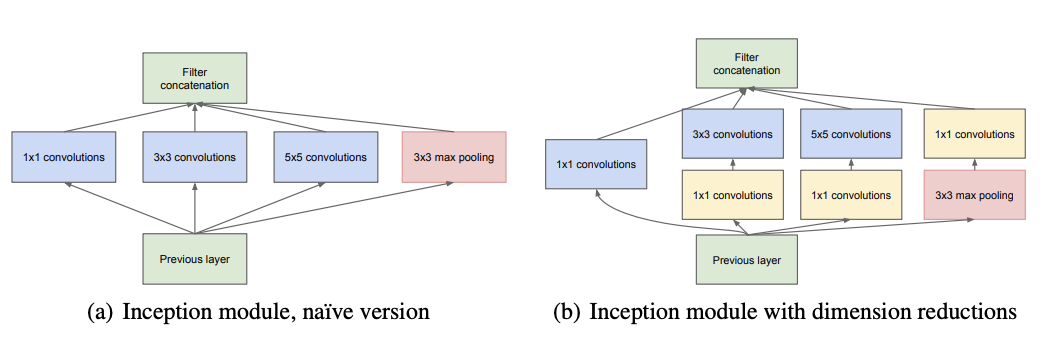Inception v2 and v3 sought to further reduce computational costs by factorizing the filters. The idea was that, the computation is more efficient when the dimensions of the input (data from the previous layer) was not significantly altered. However, too much factorization could also result in loss of information. Since the filter might not be able to accomodate all the data from the previous layer, some data will be lost or unfilterd if it lies outside the dimension of the convolution of the filter. Hence, factorization methods were used to improve computational complexity, while also producing efficient performance.

Factorization is simply reducing the convolution sizes of the filters to smaller sizes to reduce the computational cost. For example, a 5x5 convolutional node can be broken down to 2 layers of 3x3 nodes, which reduced the computational cost by a little less than 3x (2.78x for efficient to be precise). This makes the network deeper and even more so for larger dimension convolutions, and too deep of the network will eventually result in loss of information, since there will need to be more factorization as mentioned in the precious paragraph.

Another factorization method involves replacing nxn nodes with 1xn and nx1 nodes. For example, a 5x5 convolution will be replaced by a 1x5 convolution and a 5x1 convolution. This makes the network broader instead of longer (deeper) and hence reduces the chance of loss of information from layers of factorization at the same time reducing the computational cost in general. Inception v2 and v3 have the same working theory, however v3 has improvements like optimizers, 7x7 convolution factorization, label smoothing, etc.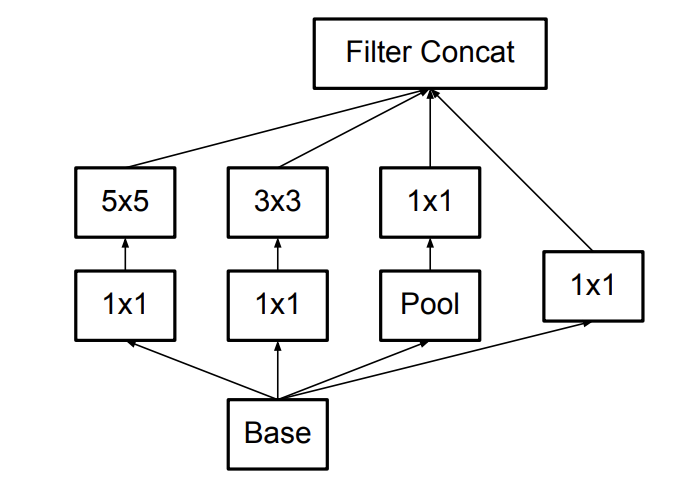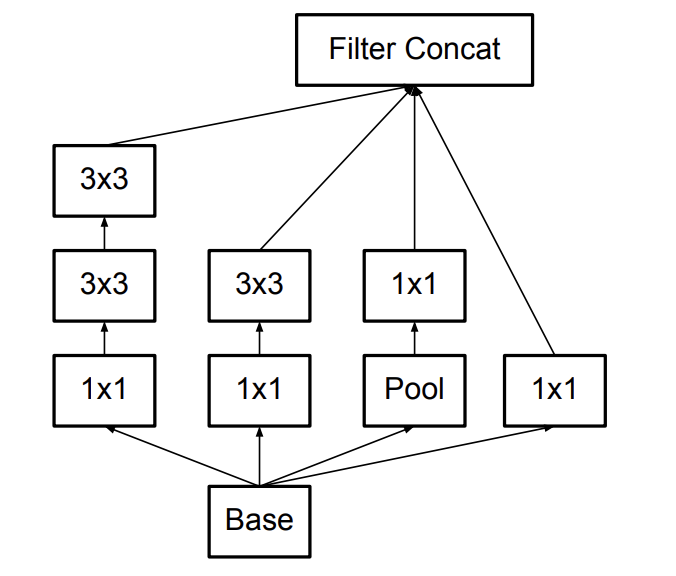Inception v2 module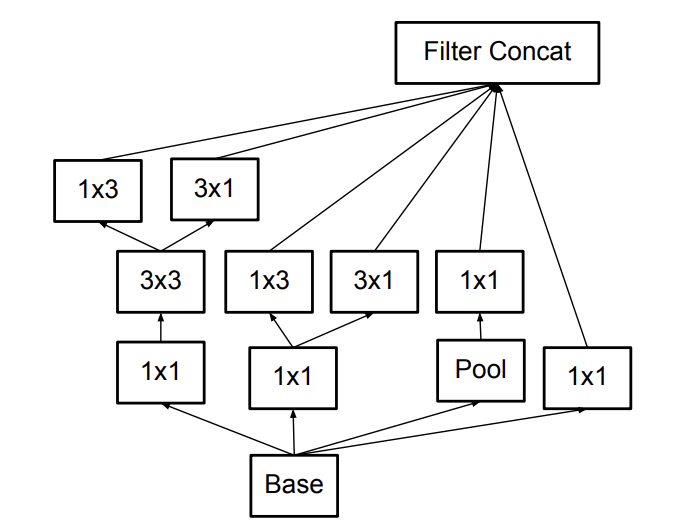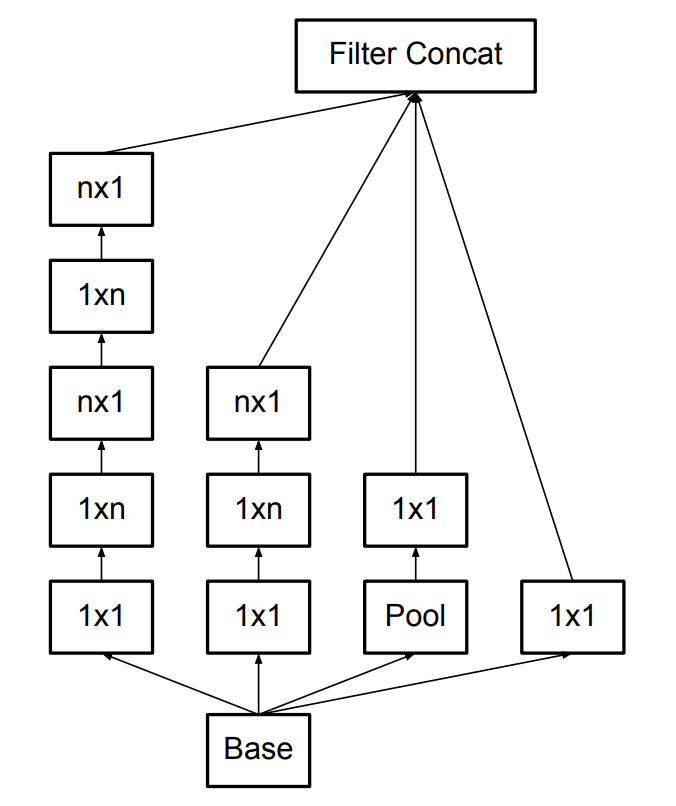1xn, nx1 factorization to make the network broader or deeper

For Inception v4 and Inception-ResNet, the idea was to eliminate unneccessary complexity by making the network more uniform. The first layer of data processing(let's call it the stem) is introduced before the Inception blocks which is designed in three different ways, namely: module A, B and C. Although Inception v2 and v3 sought to lengthen or broaden the network for more efficient performance, for Inception v4 and Inception ResNet, reduction blocks were introduced, specifically for the same functionality (to regulate the bredth and/or depth of the network).

Stem for Inception v4 and Inception-ResNet v2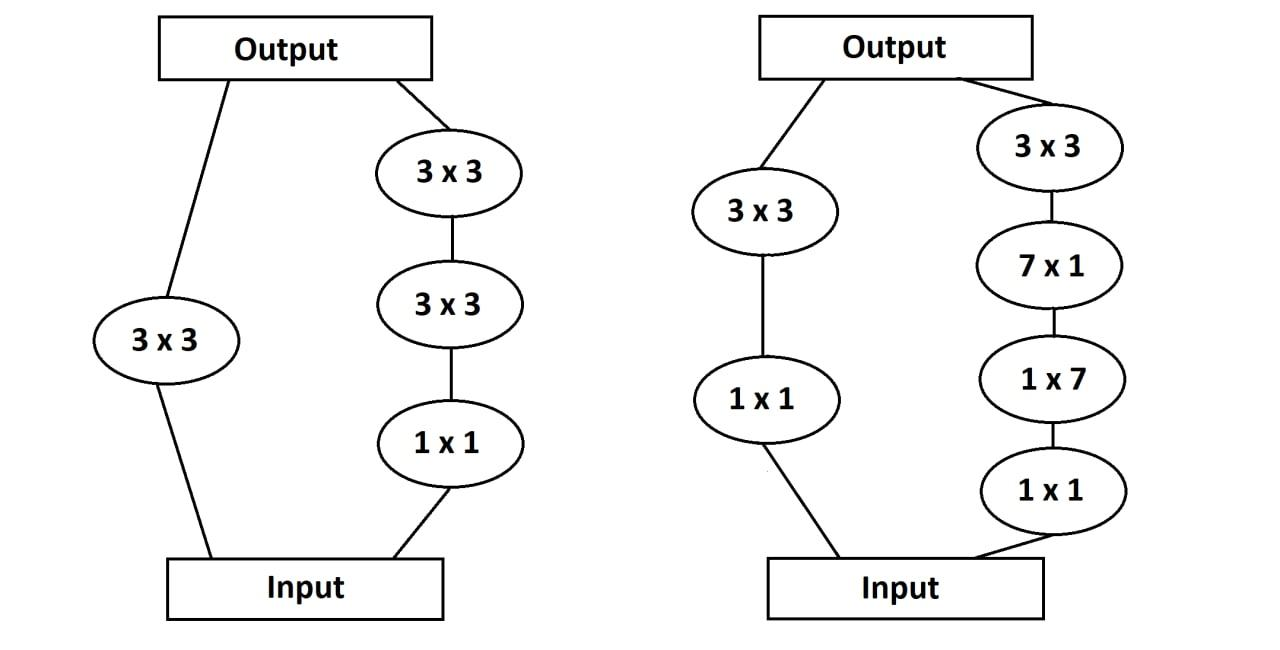Inception v4, Inception-ResNet Reduction Blocks to broaden/deepen network

# Inception-ResNet v1

The Inception-ResNet network is a hybrid network inspired both by inception and the performance of resnet. This hybrid has two versions; Inception-ResNet v1 and v2. Althought their working principles are the same, Inception-ResNet v2 is more accurate, but has a higher computational cost than the previous Inception-ResNet v1 network. In this section of the article we will focus on Inception-ResNet v1.

As stated before, the Inception-ResNet like the Inception v4 incorporates the use of 3 different stem modules and reduction blocks. However, as the this network is a hybrid between the Inception v4 and ResNet, the key functionality of the Inception-ResNet is that the output of the inception module is added to the input (i.e.data from previous layer).

For this to work, the dimensions of the output from the inception module and the input from the previous layer must have the same dimensions. Factorization hence becomes important here to match these dimensions. However, further studies showed that the network dies when the number of convolution filters exceeds 1000. Activation Scaling is then introduced and found to solve the issue of the dying network.

# Implementation of Operations in Inception-ResNet v1

In this section, I will attempt to lay out some of the different operations in the Inception-ResNet v1 model and compare to the operations in Inception and ResNet variants in terms of performance, accuracy etc, as well as listing some applications for each. I will use the TF-Slim Library from Google AI.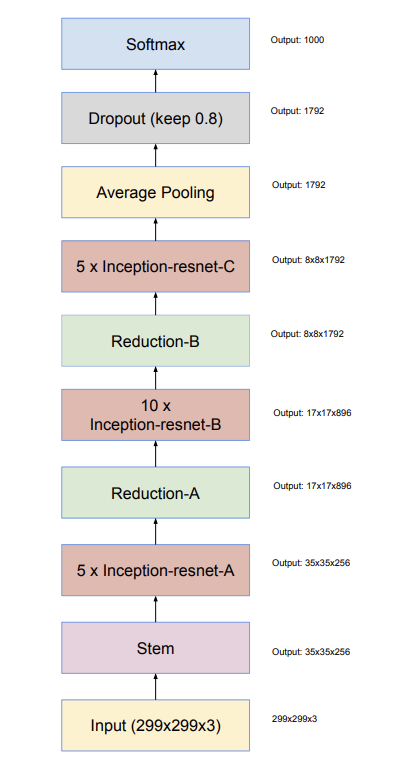This is the overall schematic for the Inception-ResNet v1 and v2 network, however the details of the components differ from each other. It is precisely these differences that we will delve into in this section.

First the Stem - find below the stems for Inception-ResNet v1(top) and v2(bottom).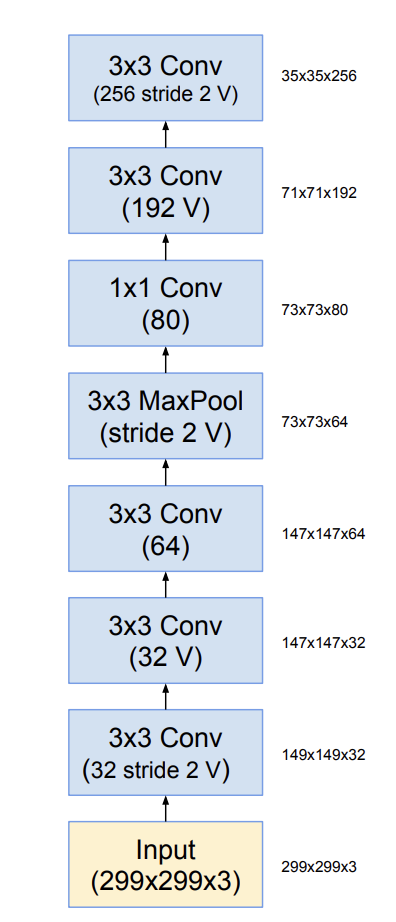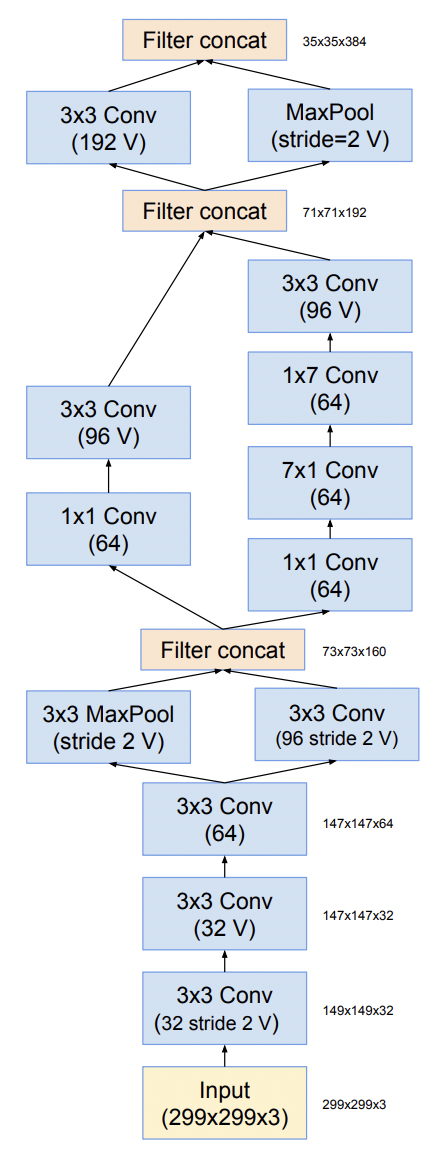Firstly, we need to import the tensorflow and tensorflow slim libraries. Now we define a function for the stem.

``````import tensorflow as tf
import tensorflow.contrib.slim as slim

def stem_v1(inputs, scope='stem_v1'):
# 149 x 149 x 32
net = slim.conv2d(inputs, 32, 3, stride=2, padding='VALID',
scope='Conv2d_1a_3x3')
end_points['Conv2d_1a_3x3'] = net
# 147 x 147 x 32
net = slim.conv2d(net, 32, 3, padding='VALID',
scope='Conv2d_2a_3x3')
end_points['Conv2d_2a_3x3'] = net
# 147 x 147 x 64
net = slim.conv2d(net, 64, 3, scope='Conv2d_2b_3x3')
end_points['Conv2d_2b_3x3'] = net
# 73 x 73 x 64
net = slim.max_pool2d(net, 3, stride=2, padding='VALID',
scope='MaxPool_3a_3x3')
end_points['MaxPool_3a_3x3'] = net
# 73 x 73 x 80
net = slim.conv2d(net, 80, 1, padding='VALID',
scope='Conv2d_3b_1x1')
end_points['Conv2d_3b_1x1'] = net
# 71 x 71 x 192
net = slim.conv2d(net, 192, 3, padding='VALID',
scope='Conv2d_4a_3x3')
end_points['Conv2d_4a_3x3'] = net
# 35 x 35 x 256
net = slim.conv2d(net, 256, 3, stride=2, padding='VALID',
scope='Conv2d_4b_3x3')
end_points['Conv2d_4b_3x3'] = net
``````

These sets of operations are quite self-explanatory, but let's define some of the parameters. Inputs is a 4-D tensor of size [batch_size, height, width, 3], note that inputs is only used as the input for the first convolution and the output for that convolution is stored in a variable called net. This net variable is updated from the output of each convolution and used as the input for the next convolution.

You can take a look at the documentation for TF-Slim library for the full definition of the convolutions and max pooling, average pooling functions. In general use cases, we only need the number of inputs, the number of outputs, convolution size, stride and scope(the padding arguement is either set to 'VALID' or 'SAME'). i.e net = slim.conv2d(input, number of outputs, convolution size, padding = 'VALID'/'SAME', scope='variable_scope').

This is the best time to ideally define all the modules and reduction blocks since we will be using them from now on. Find below, the 3 Incpetion-ResNet modules: A,B, and C (from left to right),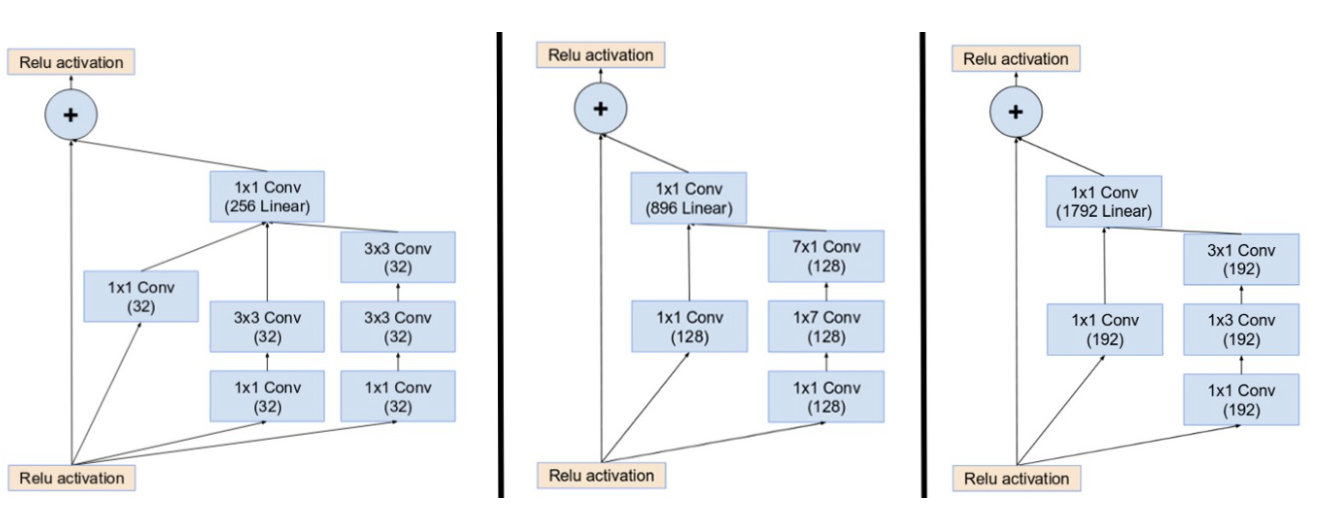and reduction blocks A and B(left and right).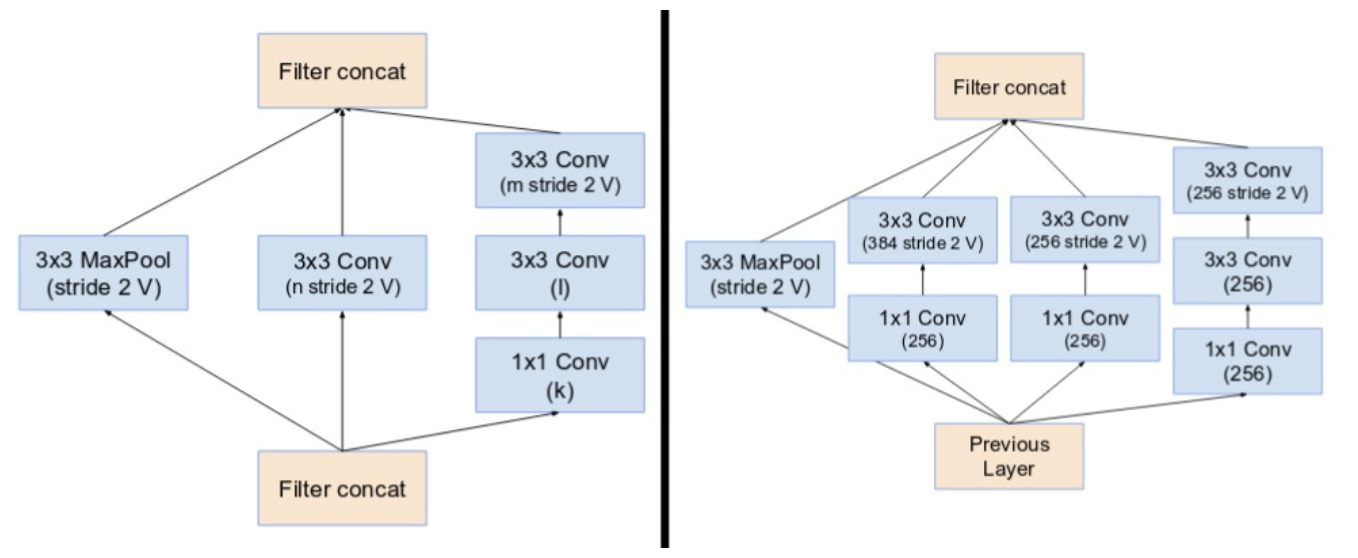``````import tensorflow as tf
import tensorflow.contrib.slim as slim
#Module-A
def block35(net, scale=1.0, activation_fn=tf.nn.relu, scope=None, reuse=None):
"""Builds the 35x35 resnet block."""
with tf.variable_scope(scope, 'Block35', [net], reuse=reuse):
with tf.variable_scope('Branch_0'):
tower_conv = slim.conv2d(net, 32, 1, scope='Conv2d_1x1')
with tf.variable_scope('Branch_1'):
tower_conv1_0 = slim.conv2d(net, 32, 1, scope='Conv2d_0a_1x1')
tower_conv1_1 = slim.conv2d(tower_conv1_0, 32, 3, scope='Conv2d_0b_3x3')
with tf.variable_scope('Branch_2'):
tower_conv2_0 = slim.conv2d(net, 32, 1, scope='Conv2d_0a_1x1')
tower_conv2_1 = slim.conv2d(tower_conv2_0, 32, 3, scope='Conv2d_0b_3x3')
tower_conv2_2 = slim.conv2d(tower_conv2_1, 32, 3, scope='Conv2d_0c_3x3')
mixed = tf.concat([tower_conv, tower_conv1_1, tower_conv2_2], 3)
up = slim.conv2d(mixed, net.get_shape(), 1, normalizer_fn=None,
activation_fn=None, scope='Conv2d_1x1')
net += scale * up
if activation_fn:
net = activation_fn(net)
return net

#Module-B
def block17(net, scale=1.0, activation_fn=tf.nn.relu, scope=None, reuse=None):
"""Builds the 17x17 resnet block."""
with tf.variable_scope(scope, 'Block17', [net], reuse=reuse):
with tf.variable_scope('Branch_0'):
tower_conv = slim.conv2d(net, 128, 1, scope='Conv2d_1x1')
with tf.variable_scope('Branch_1'):
tower_conv1_0 = slim.conv2d(net, 128, 1, scope='Conv2d_0a_1x1')
tower_conv1_1 = slim.conv2d(tower_conv1_0, 128, [1, 7],
scope='Conv2d_0b_1x7')
tower_conv1_2 = slim.conv2d(tower_conv1_1, 128, [7, 1],
scope='Conv2d_0c_7x1')
mixed = tf.concat([tower_conv, tower_conv1_2], 3)
up = slim.conv2d(mixed, net.get_shape(), 1, normalizer_fn=None,
activation_fn=None, scope='Conv2d_1x1')
net += scale * up
if activation_fn:
net = activation_fn(net)
return net

#Module-C
def block8(net, scale=1.0, activation_fn=tf.nn.relu, scope=None, reuse=None):
"""Builds the 8x8 resnet block."""
with tf.variable_scope(scope, 'Block8', [net], reuse=reuse):
with tf.variable_scope('Branch_0'):
tower_conv = slim.conv2d(net, 192, 1, scope='Conv2d_1x1')
with tf.variable_scope('Branch_1'):
tower_conv1_0 = slim.conv2d(net, 192, 1, scope='Conv2d_0a_1x1')
tower_conv1_1 = slim.conv2d(tower_conv1_0, 192, [1, 3],
scope='Conv2d_0b_1x3')
tower_conv1_2 = slim.conv2d(tower_conv1_1, 192, [3, 1],
scope='Conv2d_0c_3x1')
mixed = tf.concat([tower_conv, tower_conv1_2], 3)
up = slim.conv2d(mixed, net.get_shape(), 1, normalizer_fn=None,
activation_fn=None, scope='Conv2d_1x1')
net += scale * up
if activation_fn:
net = activation_fn(net)
return net

def reduction_a(net, k, l, m, n):
with tf.variable_scope('Branch_0'):
tower_conv = slim.conv2d(net, n, 3, stride=2, padding='VALID',
scope='Conv2d_1a_3x3')
with tf.variable_scope('Branch_1'):
tower_conv1_0 = slim.conv2d(net, k, 1, scope='Conv2d_0a_1x1')
tower_conv1_1 = slim.conv2d(tower_conv1_0, l, 3,
scope='Conv2d_0b_3x3')
tower_conv1_2 = slim.conv2d(tower_conv1_1, m, 3,
scope='Conv2d_1a_3x3')
with tf.variable_scope('Branch_2'):
tower_pool = slim.max_pool2d(net, 3, stride=2, padding='VALID',
scope='MaxPool_1a_3x3')
net = tf.concat([tower_conv, tower_conv1_2, tower_pool], 3)
return net

def reduction_b(net):
with tf.variable_scope('Branch_0'):
tower_conv = slim.conv2d(net, 256, 1, scope='Conv2d_0a_1x1')
tower_conv_1 = slim.conv2d(tower_conv, 384, 3, stride=2,
with tf.variable_scope('Branch_1'):
tower_conv1 = slim.conv2d(net, 256, 1, scope='Conv2d_0a_1x1')
tower_conv1_1 = slim.conv2d(tower_conv1, 256, 3, stride=2,
with tf.variable_scope('Branch_2'):
tower_conv2 = slim.conv2d(net, 256, 1, scope='Conv2d_0a_1x1')
tower_conv2_1 = slim.conv2d(tower_conv2, 256, 3,
scope='Conv2d_0b_3x3')
tower_conv2_2 = slim.conv2d(tower_conv2_1, 256, 3, stride=2,
with tf.variable_scope('Branch_3'):
tower_pool = slim.max_pool2d(net, 3, stride=2, padding='VALID',
scope='MaxPool_1a_3x3')
net = tf.concat([tower_conv_1, tower_conv1_1,
tower_conv2_2, tower_pool], 3)
return net
``````

Now that we have these operations, building an Inception-ResNet v1 module is simply layering these operations as outlined by the overall schematic. Note that the modules for Inception-ResNet v2 and Inception v4 have activation scaling after the incpetion network that is added before entering the ReLu function.

With this article at OpenGenus, you must have the complete idea of Inception-ResNet v1.